# 333 Results

View
Selected filters:
• Ohio Open Ed Collaborative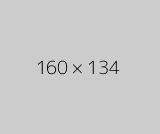Conditional Remix & Share Permitted
CC BY-NC
Rating

The Calculus I course was developed through the Ohio Department of Higher Education OER Innovation Grant. This work was completed and the course was posted in February 2019. The course is part of the Ohio Transfer Module and is also named TMM005. For more information about credit transfer between Ohio colleges and universities, please visit: www.ohiohighered.org/transfer.Team LeadJim Fowler                                         Ohio State UniversityRita Ralph                                         Columbus State Community CollegeContent ContributorsNela Lakos                                       Ohio State UniversityBart Snapp                                       Ohio State UniversityJames Talamo                                  Ohio State UniversityXiang Yan                                         Edison State Community CollegeLibrarianDaniel Dotson                                    Ohio State University                     Review TeamThomas Needham                             Ohio State UniversityCarl Stitz                                            Lakeland Community CollegeSara Rollo                                          North Central State College

Subject:
Mathematics
Calculus
Material Type:
Full Course
Provider:
Ohio Open Ed Collaborative
11/02/2020Conditional Remix & Share Permitted
CC BY-NC
Rating

After completing this section, students should be able to do the following.Compute average velocity.Approximate instantaneous velocity.Compare average and instantaneous velocity.Compute instantaneous velocity.

Subject:
Calculus
Material Type:
Module
Author:
Ohio Open Ed Collaborative
11/02/2020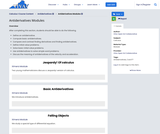Conditional Remix & Share Permitted
CC BY-NC
Rating

After completing this section, students should be able to do the following.Define an antiderivative.Compute basic antiderivatives.Compare and contrast finding derivatives and finding antiderivatives.Define initial value problems.Solve basic initial value problems.Use antiderivatives to solve simple word problems.Discuss the meaning of antiderivatives of the velocity and acceleration.

Subject:
Calculus
Material Type:
Module
Author:
Ohio Open Ed Collaborative
11/02/2020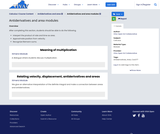Conditional Remix & Share Permitted
CC BY-NC
Rating

After completing this section, students should be able to do the following.Interpert the product of rate and time as area.Approximate position from velocity.Recognize Riemann sums.

Subject:
Calculus
Material Type:
Module
Author:
Ohio Open Ed Collaborative
11/02/2020Conditional Remix & Share Permitted
CC BY-NC
Rating

After completing this section, students should be able to do the following.Given a velocity function, calculate displacement and distance traveled.Given a velocity function, find the position function.Given an acceleration function, find the velocity function.Understand the difference between displacement and distance traveled.Understand the relationship between position, velocity and acceleration.Calculate the change in the amount.Compute the average value of the function on an interval.Understand that the average value of the function on an interval is attained by the function on that interval.

Subject:
Calculus
Material Type:
Module
Author:
Ohio Open Ed Collaborative
11/02/2020Conditional Remix & Share Permitted
CC BY-NC
Rating

After completing this section, students should be able to do the following.Identify word problems as related rates problems.Translate word problems into mathematical equations.Solve related rates word problems.

Subject:
Calculus
Material Type:
Module
Author:
Ohio Open Ed Collaborative
11/02/2020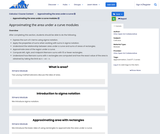Conditional Remix & Share Permitted
CC BY-NC
Rating

After completing this section, students should be able to do the following.Express the sum of n terms using sigma notation.Apply the properties of sums when working with sums in sigma notation.Understand the relationship between area under a curve and sums of areas of rectangles.Approximate area of the region under a curve.Compute left, right, and midpoint Riemann sums with 10 or fewer rectangles.Understand how Riemann sums with n rectangles are computed and how the exact value of the area is obtained by taking the limit as n→∞n→∞ .

Subject:
Calculus
Material Type:
Module
Author:
Ohio Open Ed Collaborative
11/02/2020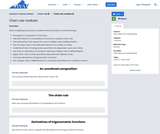Conditional Remix & Share Permitted
CC BY-NC
Rating

After completing this section, students should be able to do the following.Recognize a composition of functions.Take derivatives of compositions of functions using the chain rule.Take derivatives that require the use of multiple rules of differentiation.Use the chain rule to calculate derivatives from a table of values.Understand rate of change when quantities are dependent upon each other.Use order of operations in situations requiring multiple rules of differentiation.Apply chain rule to relate quantities expressed with different units.Compute derivatives of trigonometric functions.Use multiple rules of differentiation to calculate derivatives from a table of values.

Subject:
Calculus
Material Type:
Module
Author:
Ohio Open Ed Collaborative
11/02/2020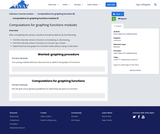Conditional Remix & Share Permitted
CC BY-NC
Rating

After completing this section, students should be able to do the following.Find the intervals where a function is increasing or decreasing.Find the intervals where a function is concave up or down.Determine how the graph of a function looks without using a calculator.

Subject:
Calculus
Material Type:
Module
Author:
Ohio Open Ed Collaborative
11/02/2020Conditional Remix & Share Permitted
CC BY-NC
Rating

After completing this section, students should be able to do the following.Understand what information the derivative gives concerning when a function is increasing or decreasing.Understand what information the second derivative gives concerning concavity of the graph of a function.Interpret limits as giving information about functions.Determine how the graph of a function looks based on an analytic description of the function.

Subject:
Calculus
Material Type:
Module
Author:
Ohio Open Ed Collaborative